## H-Atom p.1

The seemingly over-complex geometric description of motion: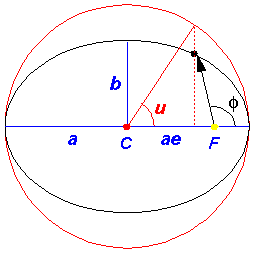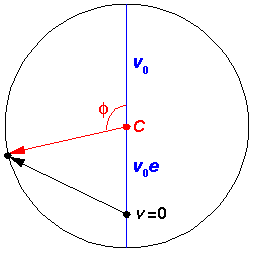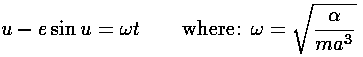can, with some work, be seen to solve the equation of motion: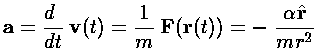Start by noting that the velocity vector is made of a constant vector (ev0j) added to the red rotating vector. Taking the time derivate removes the constant from consideration, so the acceleration vector must be tangent to the circle--i.e., rotated 90° from the red radial vector, which is itself rotated 90° from r (note that the zero for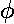was the x-axis for the orbit and the y-axis in the hodograph). Thus the acceleration vector is in the right direction: -r. The magnitude of the acceleration vector is just: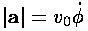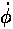is related to the (constant) angular momentum (L) [which we can evaluate for example, at the near point].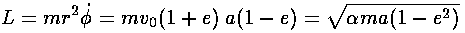Solving the above forand substituting into our equation for the magnitude of the acceleration vector, yields: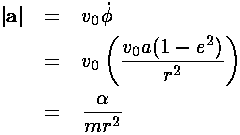Note in passing that if the angular momentum is zero, then e=1. The circular orbits (e=0) have the maximum possible angular momentum for a given semi-major axis a, that is for a given energy E because there is a simple relation between the semi-major axis a and E: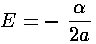Note that the energy is negative; the positive kinetic energy equals (on average) one half the absolute value of the potential energy (the Virial theorem again!) and the result is that the total energy is ½ the average potential energy.

Here is another way of presenting the results: plots displaying the position of the particle on the orbit and the velocity of the particle on the hodograph at a series of equally spaced times.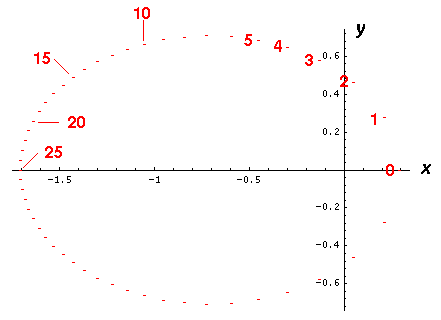The above is a plot of the (x,y) location of a electron (or planet) at successive increments of time (tics) Note the when the electron (planet) is close to the nucleus (or Sun), the particle seems to move a great distance between tics, whereas when the particle is is far from the origin, it seems to move only a little between tics. This is exactly what you would expect for a particle conserving angular momentum (i.e., following Kepler's second law of constant areal velocity).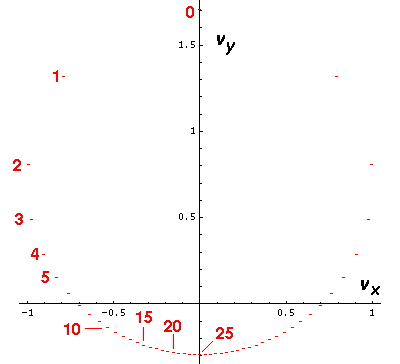The above is a plot of the (vx,vy) velocity of a electron (or planet) at successive increments of time (tics) Note the when the electron (planet) is close to the nucleus (or Sun) (e.g., near tic 0) the velocity seems to change quite a bit between tics, whereas when the particle is is far from the origin (e.g., near tic 25), the velocity seems to change only a little between tics. This is exactly what you should expect for a 1/r2 force.

Note that the motion is confined to a plane so we can display the results on a flat screen.

If we ignore the angular part of the motion, we can make plots of the the radial velocity vs. time (in tics), the distance from the origin (r) vs. time, and the speed (v) vs. time: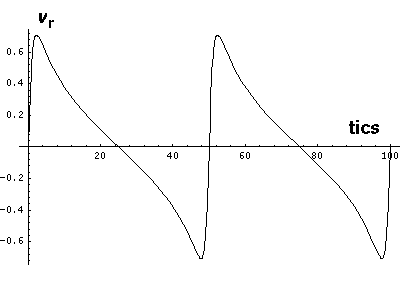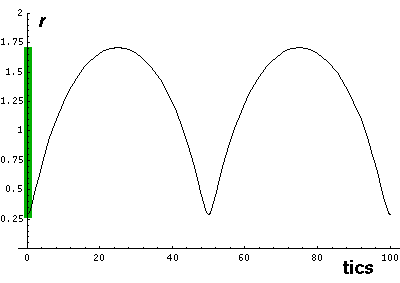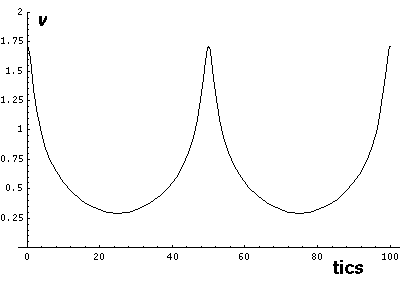Note that r seems to "bounce" off the minimum radius rmin. The motion is in fact quite similar to the bouncing falling ball. Here vr changes quickly (but not instantaneous) between negative (falling) and positive (rising) values. The green area on the r plot shows the classically allowed region.

Things happen fast during the "bounce" when the electron is near the nucleus, i.e., when the forces are large. Here are the details: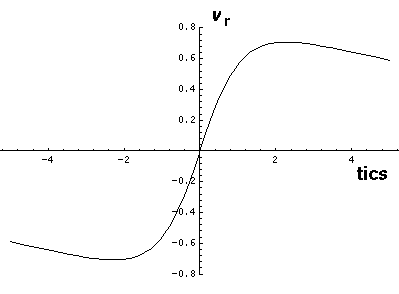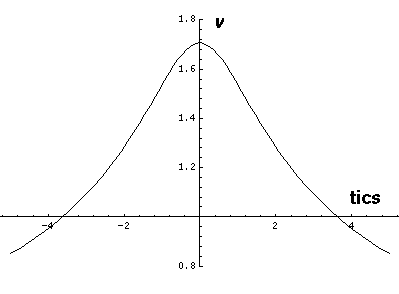Note that while r does range between two values: rmin= a(1-e) & rmax=a(1+e), these turning points are a bit different from our 1-d turning points as v is never zero. We can, however, threat our 3-d (reduced to 2-d) motion as a 1-d motion in an effective potential (see for example, Marion p.301, Barger & Olsson p.138, Goldstein p.61) which includes the centrifugal potential:The above diagram plots various the energies involved as a function of r. The line representing "Total Energy" is flat, because total energy is conserved (i.e., is constant). Total energy is the sum of kinetic energy and potential energy. We can decompose kinetic energy (½mv2) into two pieces by using the Pythagorean theorem to decompose v2 into the sum of two terms: radial velocity squared (vr2) and the component of velocity perpendicular to r ("transverse" velocity) squared. The "transverse" kinetic energy, can be calculated from the angular momentum and r...the result is the centrifugal potential. The centrifugal potential has been added to the 1/r potential; the result is plotted in red. Thus total energy is the sum of the effective potential and the "radial" kinetic energy. At the turning points there is zero "radial" kinetic energy, and the radial velocity is zero (i.e., the particle is no longer moving in or out: r is either at a minimum or a maximum). We note that for orbital motion, the time to complete a radial oscillation :

(i.e., rminrmaxrmin) is also the time it takes to go around the Sun (i.e., forto increase by 2). [In the case of the 2-d oscillator, the radial period was one half theperiod. In general there is no relationship between the two periods; as a result the orbit is not a closed figure.]